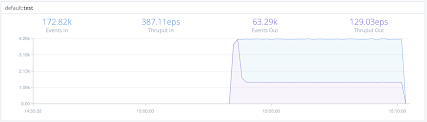# Dynamic Sampling

## Description

The Dynamic Sampling Function filters out events based on an expression, a sample mode, and events' volume. Your sample mode’s configuration determines what percentage of incoming events will be passed along to the next step.

## Usage

Filter: Filter expression (JS) that selects data to be fed through the Function. Defaults to `true`, meaning that all events passed into the Function will be evaluated.

Final: If true, stops data from being fed to the downstream Functions. Defaults to `No`.

Sample mode: Defines how sample rate will be derived. For formulas and usage details, see Sample Modes below. Supported methods:

• Logarithmic (the default): `log(previousPeriodCount)`.
• Square root: `sqrt(previousPeriodCount)`.

Sample group key: Expression used to derive sample group key. For example: `\${domain}:\${httpCode}`. Each sample group will have its own derived sampling rate, based on volume. Defaults to ``\${host}``.

All events without a host field passing through the Function will be associated with the same group and sampled the same.

• Sample period Sec: How often (in seconds) sample rates will be adjusted. Defaults to `30`.

• Minimum events: Minimum number of events that must be received, in previous sample period, for sampling mode to be applied to current period. If the number of events received for a sample group is less than this minimum, a sample rate of 1:1 is used. Defaults to `30`.

• Max sampling rate. Maximum sampling rate. If the computed sampling rate is above this value, the rate will be limited to this value.

## How Does Dynamic Sampling Work

Compared to static sampling, where users must select a sample rate a priori, Dynamic Sampling allows for automatically adjusting sampling rates, based on incoming data volume per sample group. This Function allows users to set only the aggressiveness/coarseness of this adjustment. Square Root is more aggressive than Logarithmic mode.

As an event passes through the Function, it's evaluated against the Sample Group Key expression to determine the sample group it will be associated with. For example, given an event with these fields: `...ip=1.2.3.42, port=1234...`, and a Sample Group Key of ``\${ip}:\${port}``, the event will be associated with the `1.2.3.42:1234` sample group.

### 🚧

If the Sample Group Key is left at its ``\${host}`` default, all events without a host will be associated with the same group and sampled the same.

When a sample group is new, it will initially have a sample rate of `1:1` for `Sample Period` seconds (this value defaults to 30 seconds). Once `Sample Period` seconds have elapsed, a sample rate will be derived based on the configured `Sample Mode`, using the sample group's event volume during the previous sample period.

For example, assuming a Logarithmic Sample Mode:

Period 0 (first 30s): Number of events in sample group: `1000`, Sample Rate: `1:1`, Events allowed: `ALL`
Sample Rate calculation for next period: `Math.ceil(Math.log(1000)) = 7`

Period 1 (next 30s) -- Number of events in sample group: `4000`, Sample Rate: `7:1`: Events allowed: `572`
Sample Rate calculation for next period: `Math.ceil(Math.log(4000)) = 9`

Period 2 (next 30s) -- Number of events in sample group: `12000`, Sample Rate: `9:1`: Events allowed: `1334`
Sample Rate calculation for next period: `Math.ceil(Math.log(12000)) = 10`

Period 3 (next 30s) -- Number of events in sample group: `2000`, Sample Rate: `10:1`: Events allowed: `200`
Sample Rate calculation for next period: `Math.ceil(Math.log(2000)) = 8`
...

### Sample Modes

1. Logarithmic – The sample rate is derived, for each sample group, using a natural log: `Math.ceil(Math.log(lastPeriodVolume))`. This mode is less aggressive, and drops fewer events.

2. Square Root – The sample rate is derived, for each sample group, using: `Math.ceil(Math.sqrt(lastPeriodVolume))`. This mode is more aggressive, and drops more events.

## Example

Here’s an example that illustrates the effectiveness of using the Square Root sample mode.

### Settings:

Sample Mode: `Square Root`
Sample Period (sec): `20`
Minimum Events: `3`
Max. Sampling Rate: `3`

### Results:

Events In: 4.23K
Events Out: 1.41KIn this generic example, we reduced the incoming event volume from 4.23K to 1.41K. Your own results will vary depending on multiple parameters – the Sample Group Key, Sample Period, Minimum Events, Max Sampling Rate, and rate of incoming events.

### 📘

For further examples, see Getting Smart and Practical With Dynamic Sampling.Updated 6 days ago

# Dynamic Sampling

### Suggested Edits are limited on API Reference Pages

You can only suggest edits to Markdown body content, but not to the API spec.